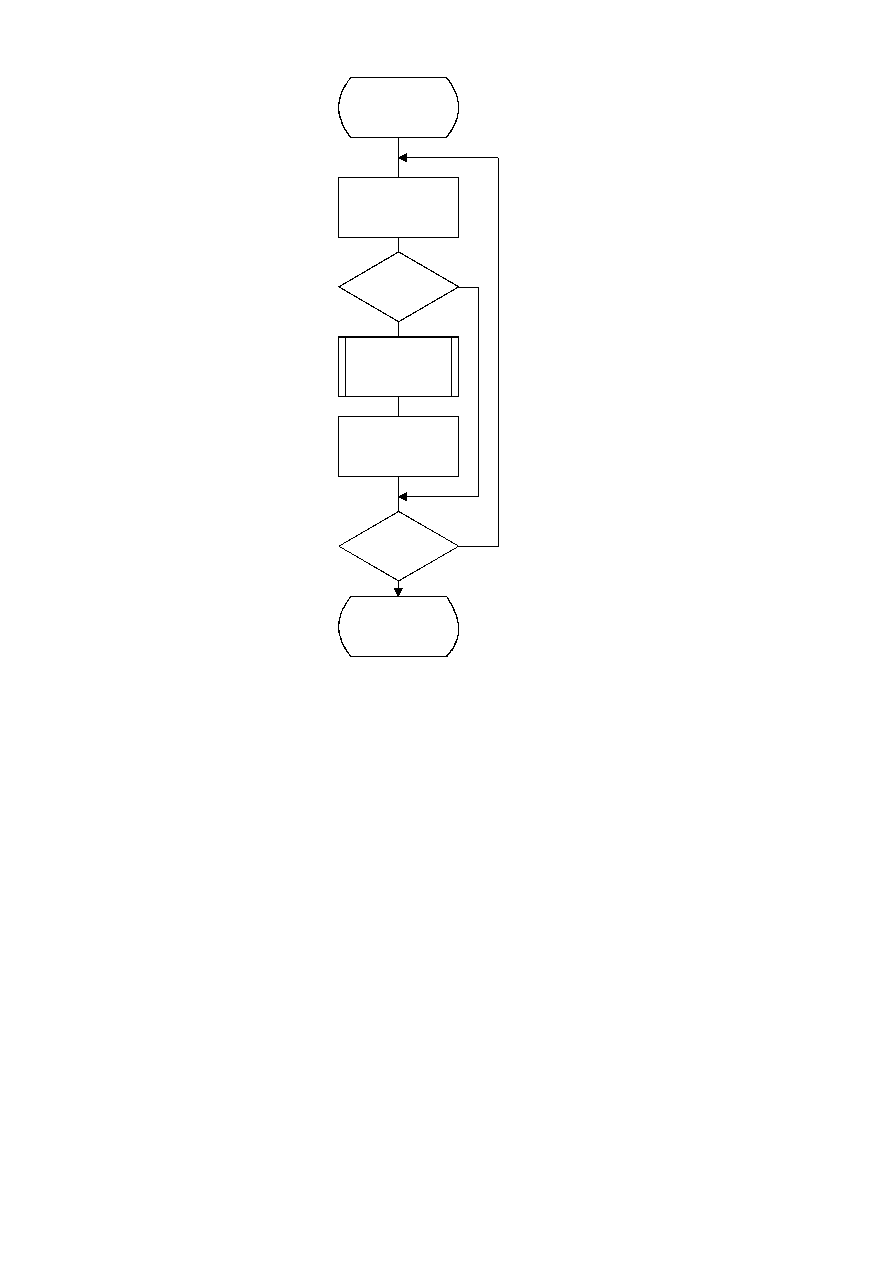ISO/IEC 10918-1 : 1993(E)
TISO1080-93/d045
Renorm_e
A = SLL A 1
C = SLL C 1
CT = CT ­ 1
No
Yes
Done
CT = 8
Byte_out
No
Yes
A < X'8000'
?
CT = 0
?
Figure D.7 ­ Encoder renormalization procedure
Figure D.7 [D45], = 16.5 cm = 645.%
The Byte_out procedure used in Renorm_e is shown in Figure D.8. This procedure uses byte-stuffing procedures which
prevent accidental generation of markers by the arithmetic encoding procedures. It also includes an example of a
procedure for resolving carry-over. For simplicity of exposition, the buffer holding the entropy-coded segment is assumed
to be large enough to contain the entire segment.
In Figure D.8 BP is the entropy-coded segment pointer and B is the compressed data byte pointed to by BP. T in Byte_out
is a temporary variable which is used to hold the output byte and carry bit. ST is the stack counter which is used to count
X'FF' output bytes until any carry-over through the X'FF' sequence has been resolved. The value of ST rarely exceeds 3.
However, since the upper limit for the value of ST is bounded only by the total entropy-coded segment size, a precision of
32 bits is recommended for ST.
Since large values of ST represent a latent output of compressed data, the following procedure may be needed in high
speed synchronous encoding systems for handling the burst of output data which occurs when the carry is resolved.
CCITT Rec. T.81 (1992 E)
63